Courses

Test: Single Correct MCQs: Waves | JEE Advanced

26 Questions MCQ Test Physics 35 Years JEE Main & Advanced Past year Papers | Test: Single Correct MCQs: Waves | JEE Advanced

Description
Attempt Test: Single Correct MCQs: Waves | JEE Advanced | 26 questions in 50 minutes | Mock test for JEE preparation | Free important questions MCQ to study Physics 35 Years JEE Main & Advanced Past year Papers for JEE Exam | Download free PDF with solutions
QUESTION: 1

A cylindrical tube open at both ends, has a fundamental frequency ‘f’ in air. The tube is dipped vertically in air. The tube is dipped vertically in water so that half of it is in water. The fundamental frequency of the air column in now

Solution: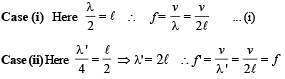QUESTION: 2

A wave represented by the equation y = a cos (kx - ωt) is superposed with another wave to form a stationary wave such that point x = 0 is a node. The equation for the other wave is

Solution:

NOTE :  Stationary wave is produced when two waves travel in opposite direction.
Now, y = a cos (kx – ωt) – a cos (kx + wt)
∴ y = 2a sin kx sin ωt is equation of stationary wave which gives a node at x = 0.

QUESTION: 3

An object of specific gravity ρ is hung from a thin steel wire. The fundamental frequency for transverse standing waves in the wire is 300 Hz. The object is immersed in water so that one half of its volume is submerged. The new fundamental frequency in Hz is

Solution:

In air : T = mg = ρ Vg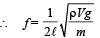... (i)

In water : T = mg – upthrust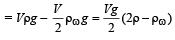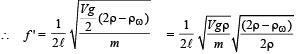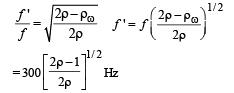QUESTION: 4

A wave disturbance in a medium is described by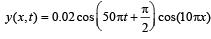where x and y are in metre and t is in second

Solution:

Comparing it with

y (x, t) = A cos (ωt + π/2) cos k x
If k x = π/2, a node occurs;
∴ 10π x = π/2 ⇒ x = 0.05 m
If k x = π, an antinode occurs
⇒ 10πx = π ⇒ x = 0.1 m

Also speed of wave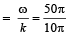= 5m/s and λ = 2π/k  = 2π/10π = 0.2 m

QUESTION: 5

The extension in a string, obeying Hooke’s law, is x. The speed of sound in the stretched string is v. If the extension in the string is increased to 1.5x, the speed of sound will be

Solution:

Velocity of sound by a stretched string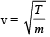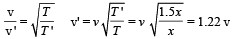QUESTION: 6

An open pipe is suddenly closed at one end with the result that the frequency of third harmonic of the closed pipe is found to be higher by 100Hz than the fundamental frequency of the open pipe. The fundamental frequency of the open pipe is

Solution:

For both  end open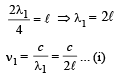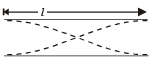For one end closed

For third harmonic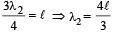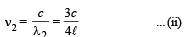Given  n2 – n1 = 100
From (i) and (ii)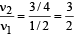On solving, we get   v1 = 200 Hz.

QUESTION: 7

A travelling wave in a stretched string is described by the equation y = A sin (kx –ωt) The maximum particle velocity is

Solution: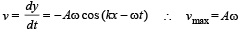QUESTION: 8

A train moves towards a stationary observer with speed 34 m/s. The train sounds a whistle and its frequency registered by the observer is f1. If the train's speed is reduced to 17 m/s, the frequency registered is f2. If the speed of sound is 340 m/s, then the ratio f1/f2 is

Solution: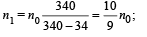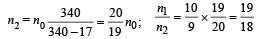QUESTION: 9

Two vibrating strings of the same material but lengths L and 2L have radii 2r and r respectively. They are stretched under the same tension. Both the strings vibrate in their fundamental nodes, the one of length L with frequency v1 and the other with frequency v2. The raio v1/v2 is given by

Solution: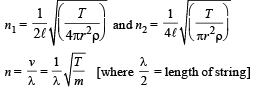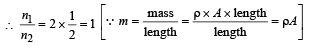QUESTION: 10

Two monatomic ideal gases 1 and 2 of molecular masses m1 and m2  respectively are enclosed in separate containers kept at the same temperature. The ratio of the speed of sound in gas 1 to that in gas 2 is given by

Solution: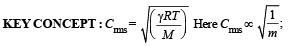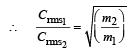QUESTION: 11

Two pulses in a stretched string whose centers are initially 8 cm apart are moving towards each other as shown in the figure. The speed of each pulse is 2 cm/s. After 2 seconds, the total energy of the pulses will be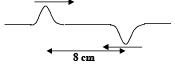Solution: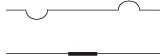After two seconds pulses will overlap each other.
NOTE : According to superposition principle the string will not have any distortion and will be straight.
Hence there will be no P.E. The total energy will be only kinetic.

QUESTION: 12

The ends of a stretched wire of length L are fixed at x = 0 and x = L. In one experiment, the displacement of the wire is y1 = A sin (πx/L) sin ωt and energy is E1 and in another experiment its displacement is y2 = A sin (2πx/L) sin 2ωt and energy is E2. Then

Solution:

E ∝ A2n2 where A = amplitude and n = frequency.
Also ω = 2πn ⇒ ω ∝ v
In case 1 : Amplitude = A and n1 = v
In case 2 : Amplitude = A and v2 = 2v

QUESTION: 13

A siren placed at a railway platform is emitting sound of frequency 5 kHz. A passenger sitting in a moving train A records a frequency of 5.5 kHz while the train approaches the siren. During his return journey in a different train B he records a frequency of 6.0 kHz while approaching the same siren. The ratio of the velocity of train B to that train A is

Solution: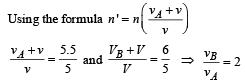QUESTION: 14

A sonometer wire resonates with a given tuning fork forming standing waves with five antinodes between the two bridges when a mass of 9 kg is suspended from the wire. When this mass is replaced by a mass M, the wire resonates with the same tuning fork forming three antinodes for the same positions of the bridges. The value of M is

Solution: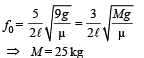⇒ M = 25 kg

NOTE : Using the formula of a vibrating string,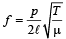where p = number of loops.
In each case, the wire vibrates, in resonance with the same tuning fork. Frequency of wire remains same while p and T change.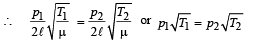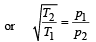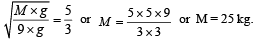QUESTION: 15

A police car moving at 22 m/s, chases a motorcyclist. The police man sounds his horn at 176 Hz, while both of them move towards a stationary siren of frequency 165 Hz.
Calculate the speed of the motorcycle, if it is given that he does not observes any beats.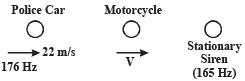Solution:

f1= frequency of the police car heard by motorcyclist,
f2 = frequency of the siren heard by motorcyclist.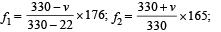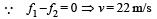QUESTION: 16

In the experiment for the determination of the speed of sound in air using the resonance column method, the length of the air column that resonates in the fundamental mode, with a tuning fork is 0.1 m. When this length is changed to 0.35 m, the same tuning fork resonates with the first overtone.
Calculate the end correction.

Solution: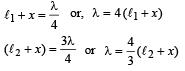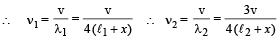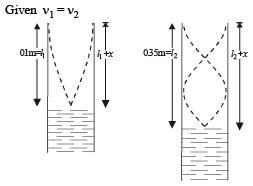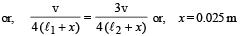QUESTION: 17

A pipe of length ℓ1, closed at one end is kept in a chamber of gas of density ρ1. A second pipe open at both ends is placed in a second chamber of gas of density ρ2. The compressibility of both the gases is equal. Calculate the length of the second pipe if frequency of first overtone in both the cases is equal

Solution:

Frequency of first overtone in closed pipe,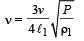.. (i)

Frequency of first overtone in open pipe,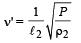... (ii)

From equation (i) and (ii)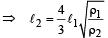QUESTION: 18

In a resonance tube with tuning fork of frequency 512 Hz, first resonance occurs at water level equal to 30.3 cm and second resonance occurs at 63.7 cm. The maximum possible error in the speed of sound is

Solution: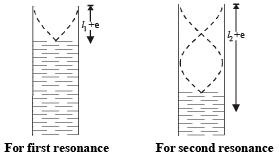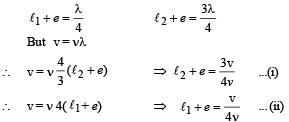Subtracting (i) and (ii),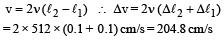QUESTION: 19

An open pipe is in resonance in 2nd harmonic with frequency f1. Now one end of the tube is closed and frequency is increased to f2 such that the resonance again occurs in nth harmonic. Choose the correct option

Solution: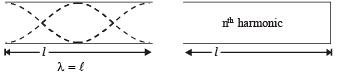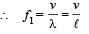... (i)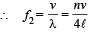... (ii)

Here n is a odd number. From (i) and (ii)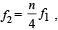For first resonance,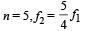QUESTION: 20

A massless rod of length L is suspended by two identical strings AB and CD of equal length. A block of mass m is suspended from point O such that BO is equal to ‘x’. Further it is observed that the frequency of 1st harmonic in AB is equal to 2nd harmonic frequency in CD. ‘x’ is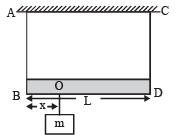Solution:

Frequency of Ist harmonic of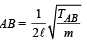Frequency of 2nd harmonic of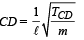Given that the two frequencies are equal.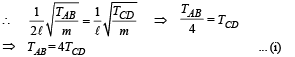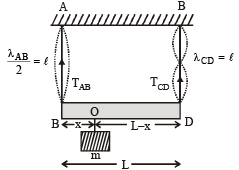For rotational equilibrium of massless rod, taking torque about point O.
TAB × x = TCD (L – x) ... (ii)
For translational equilibrium,

TAB + TCD = mg ... (iii)

On solving, (i) and (iii), we get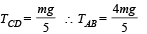Substituting these values in (ii), we get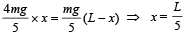QUESTION: 21

In the experiment to determine the speed of sound using a resonance column,

Solution:

As shown in the figure, the fringes of the tuning fork are kept in a vertical plane.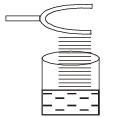QUESTION: 22

A transverse sinusoidal wave moves along a string in the positive x-direction at a speed of 10 cm/s. The wavelength of the wave is 0.5 m and its amplitude is 10 cm. At a particular time t, the snap–shot of the wave is shown in figure. The velocity of point P when its displacement is 5 cm is –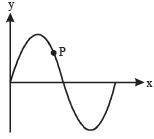Solution:

Since the wave is sinusoidal moving in positive x-axis the point will move parallel to y-axis therefore options (c) and (d) are ruled out. As the wave moves forward in positive X-direction, the point should move upwards i.e. in the positive Y-direction. Therefore correct option is a.

QUESTION: 23

A vibrating string of certain length ℓ under a tension T resonates with a mode corresponding to the first overtone (third harmonic) of an air column of length 75 cm inside a tube closed at one end. The string also generates 4 beats per second when excited along with a tuning fork of frequency n. Now when the tension of the string is slightly increased the number of beats reduces 2 per second.
Assuming the velocity of sound in air to be 340 m/s, the frequency n of the tuning fork in Hz is

Solution:

The frequency (v) produced by the air column is given by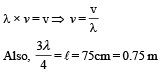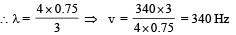∴ The frequency of vibrating string = 340. Since this string produces 4 beats/sec with a tuning fork of frequency n therefore n = 340 + 4 or n = 340 – 4. With increase in tension, the frequency produced by string increases. As the beats/sec decreases therefore n = 340 + 4 = 344 Hz.

QUESTION: 24

A hollow pipe of length 0.8 m is closed at one end. At its open end a 0.5 m long uniform string is vibrating in its second harmonic and it resonates with the fundamental frequency of the pipe. If the tension in the wire is 50 N and the speed of sound is 320 ms–1, the mass of the string is

Solution:

Frequency of 2nd harmonic of string = Fundamental frequency produced in the pipe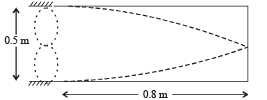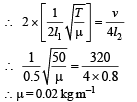The mass of the string = μ l1
= 0.02 × 0.5 kg = 10g

QUESTION: 25

A police car with a siren of frequency 8 kHz is moving with uniform velocity 36 km/hr towards a tall building which reflects the sound waves. The speed of sound in air is 320 m/s. The frequency of the siren heard by the car driver is

Solution: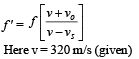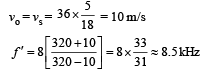QUESTION: 26

A student is performing the experiment of resonance column. The diameter of the column tube is 4 cm. The frequency of the tuning fork is 512 Hz. The air temperature is 38°C in which the speed of sound is 336 m/s. The zero of the meter scale coincides with the top end of the resonance column tube. When the first resonance occurs, the reading of the water level in the column is

Solution:

Considering the end correction [e = 0.3 D], we get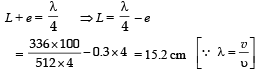Use Code STAYHOME200 and get INR 200 additional OFF Use Coupon Code

Track your progress, build streaks, highlight & save important lessons and more!

Similar ContentRelated tests